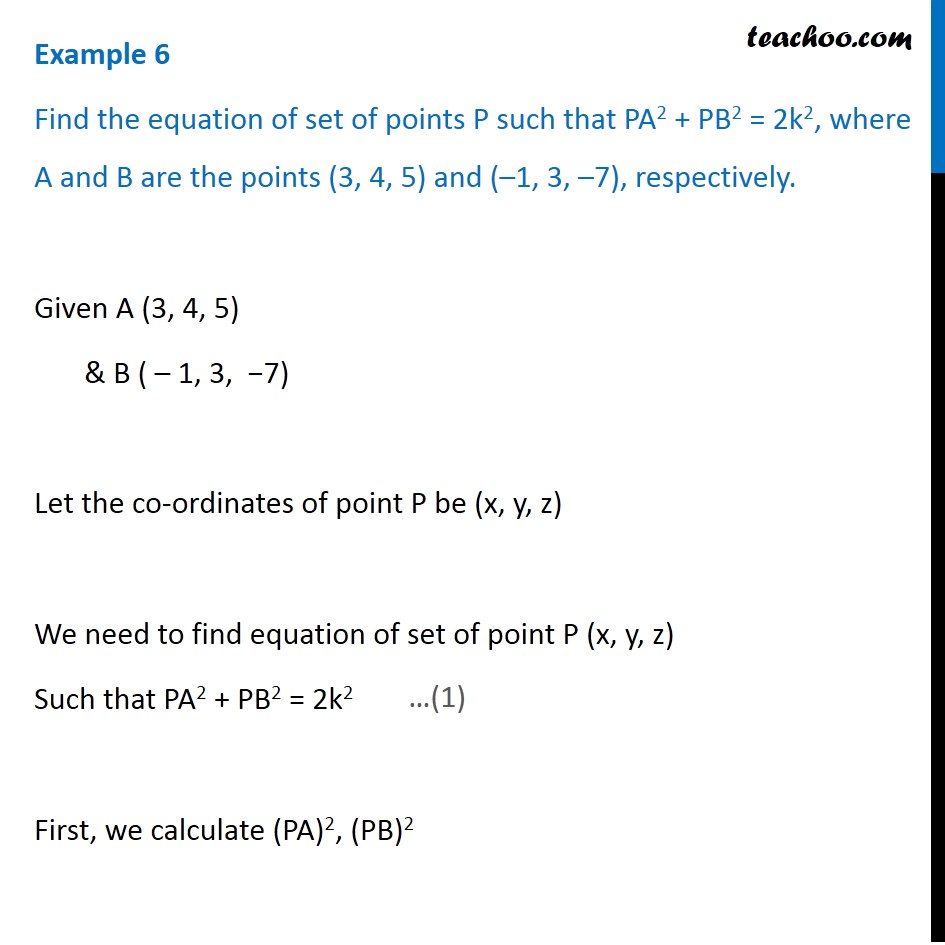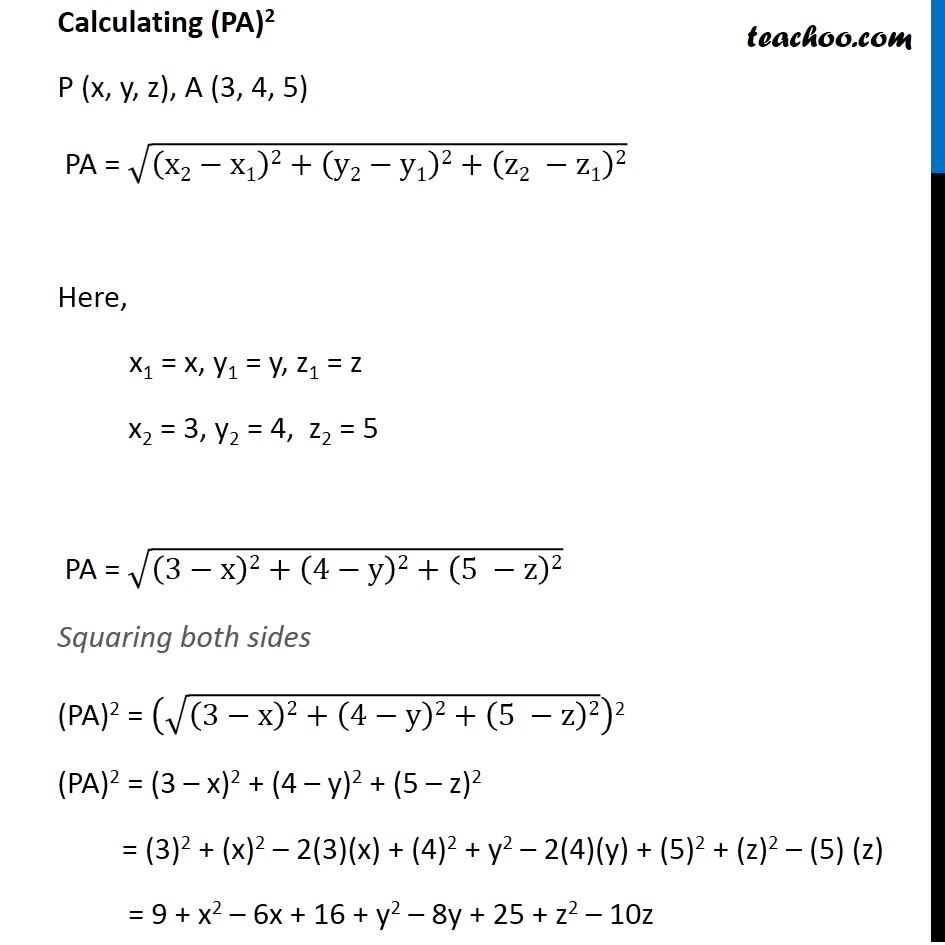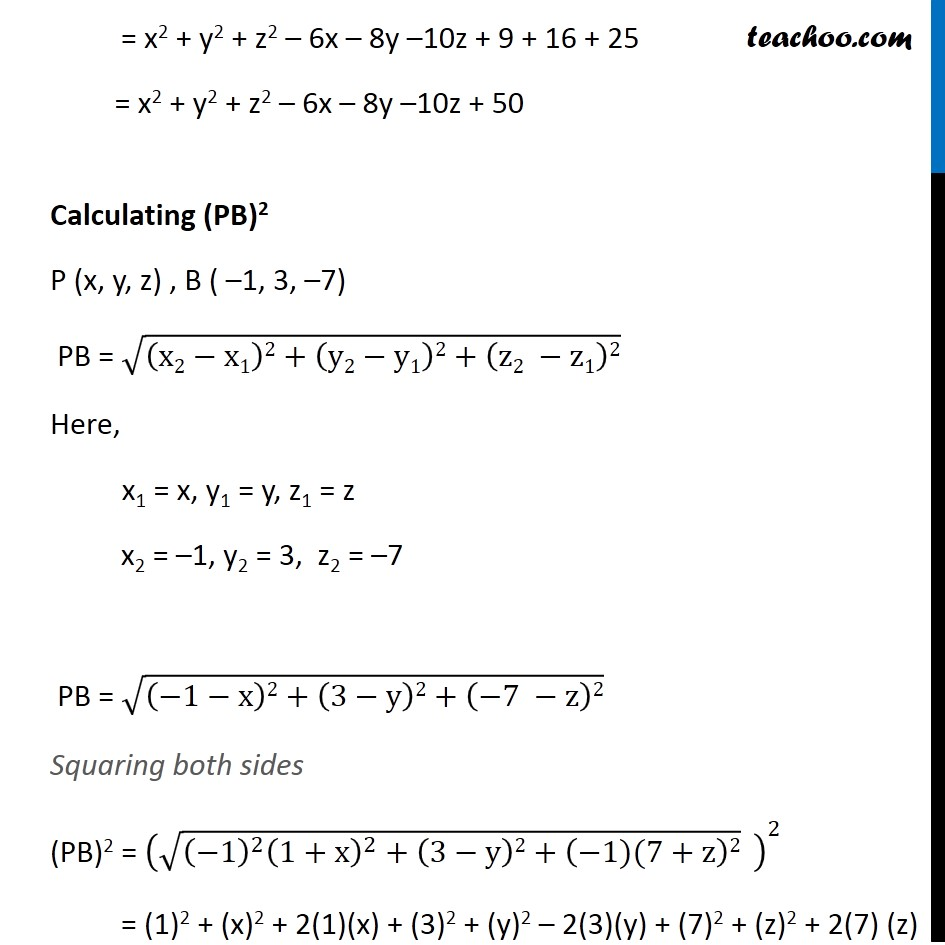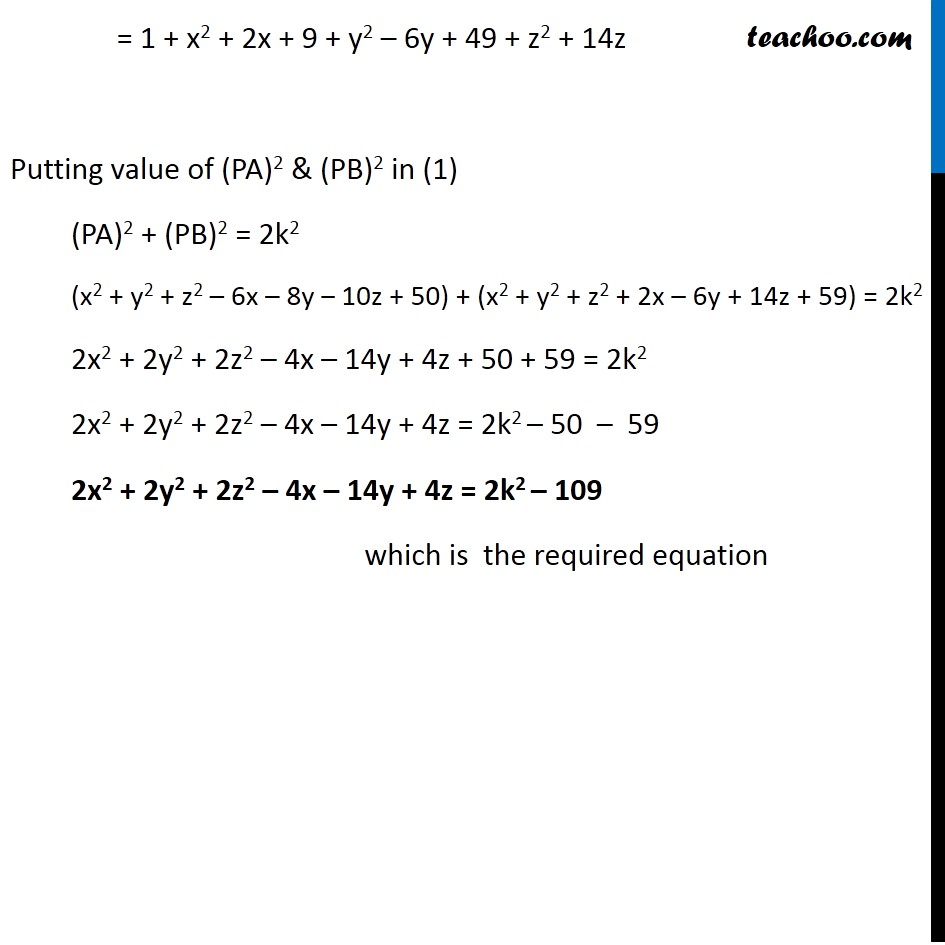Examples

Chapter 11 Class 11 - Intro to Three Dimensional Geometry
Serial order wiseLearn in your speed, with individual attention - Teachoo Maths 1-on-1 Class

### Transcript

Example 6 Find the equation of set of points P such that PA2 + PB2 = 2k2, where A and B are the points (3, 4, 5) and (–1, 3, –7), respectively. Given A (3, 4, 5) & B ( – 1, 3, −7) Let the co-ordinates of point P be (x, y, z) We need to find equation of set of point P (x, y, z) Such that PA2 + PB2 = 2k2 First, we calculate (PA)2, (PB)2 Calculating (PA)2 P (x, y, z), A (3, 4, 5) PA = √((x2−x1)2+(y2−y1)2+(z2 −z1)2) Here, x1 = x, y1 = y, z1 = z x2 = 3, y2 = 4, z2 = 5 PA = √((3−x)2+(4−y)2+(5 −z)2) Squaring both sides (PA)2 = (√((3−x)2+(4−y)2+(5 −z)2))2 (PA)2 = (3 – x)2 + (4 – y)2 + (5 – z)2 = (3)2 + (x)2 – 2(3)(x) + (4)2 + y2 – 2(4)(y) + (5)2 + (z)2 – (5) (z) = 9 + x2 – 6x + 16 + y2 – 8y + 25 + z2 – 10z = x2 + y2 + z2 – 6x – 8y –10z + 9 + 16 + 25 = x2 + y2 + z2 – 6x – 8y –10z + 50 Calculating (PB)2 P (x, y, z) , B ( –1, 3, –7) PB = √((x2−x1)2+(y2−y1)2+(z2 −z1)2) Here, x1 = x, y1 = y, z1 = z x2 = –1, y2 = 3, z2 = –7 PB = √((−1−x)2+(3−y)2+(−7 −z)2) Squaring both sides (PB)2 = (√((−1)^2 (1+x)^2+(3−y)2+(−1)(7+z)2) " " )^2 = (1)2 + (x)2 + 2(1)(x) + (3)2 + (y)2 – 2(3)(y) + (7)2 + (z)2 + 2(7) (z) = 1 + x2 + 2x + 9 + y2 – 6y + 49 + z2 + 14z Putting value of (PA)2 & (PB)2 in (1) (PA)2 + (PB)2 = 2k2 (x2 + y2 + z2 – 6x – 8y – 10z + 50) + (x2 + y2 + z2 + 2x – 6y + 14z + 59) = 2k2 2x2 + 2y2 + 2z2 – 4x – 14y + 4z + 50 + 59 = 2k2 2x2 + 2y2 + 2z2 – 4x – 14y + 4z = 2k2 – 50 – 59 2x2 + 2y2 + 2z2 – 4x – 14y + 4z = 2k2 – 109 which is the required equation